SLVA689 February   2015

1. I2CBus Pullup Resistor Calculation

### 2 Pullup Resistor Calculation

A strong pullup (small resistor) prevents the I2C pin on an IC from being able to drive low. The VOL level that can be read as a valid logical low by the input buffers of an IC determines the minimum pullup resistance [RP(min)]. RP(min) is a function of VCC, VOL (max), and IOL:

Equation 1.The maximum pullup resistance is limited by the bus capacitance (Cb) due to I2C standard rise time specifications. If the pullup resistor value is too high, the I2C line may not rise to a logical high before it is pulled low. The response of an RC circuit to a voltage step of amplitude VCC, starting at time t = 0 is characterized by time constant RC. The voltage waveform can be written as:

Equation 2.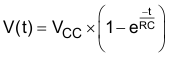For VIH = 0.7 × VCC:

Equation 3.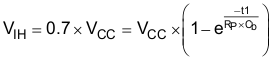For VIL = 0.3 × VCC:

Equation 4.The rise time for the I2C bus can be written as:

Equation 5.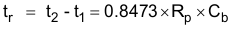The maximum pullup resistance is a function of the maximum rise time (tr):

Equation 6.where parametrics from I2C specifications are listed in Table 1.

### Table 1. Parametrics from I2C specifications

Parameter Standard Mode
(Max)
Fast Mode
(Max)
Fast Mode Plus
(Max)
Unit
tr Rise time of both SDA and SCL signals 1000 300 120 ns
Cb Capacitive load for each bus line 400 400 550 pF
VOL Low-level output voltage (at 3 mA current sink, VCC > 2 V) 0.4 0.4 0.4 V
Low-level output voltage (at 2 mA current sink, VCC ≤ 2 V) 0.2 × VCC 0.2 × VCC V

The RP (min) is plotted as a function of VCC in Figure 2. The RP (max) is plotted as a function of Cb in Figure 3 for standard-mode and fast-mode I2C.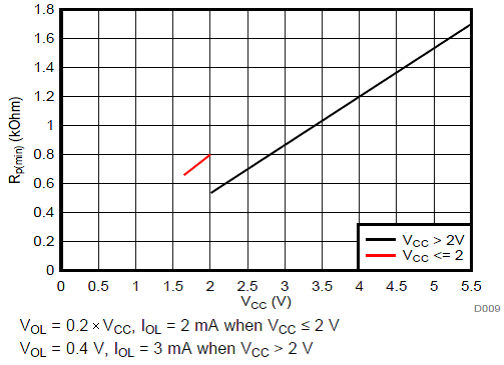Figure 2. Minimum Pullup Resistance [RP (min)] vs Pullup Reference Voltage (VCC)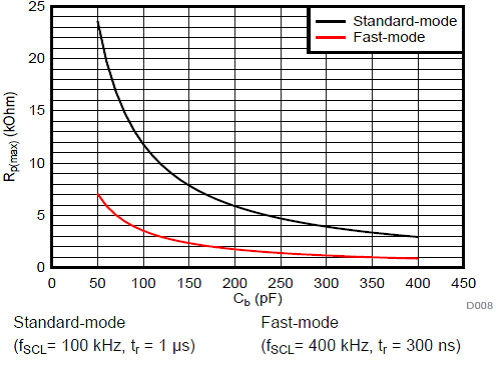Figure 3. Maximum Pullup Resistance [RP (max)] vs Bus Capacitance (Cb)